• 基于矩阵分解的协同过滤的电影推荐系统，Python实现。AI
• 利用基于矩阵分解的协同过滤算法实现电影推荐系统基于矩阵分解的协同过滤算法基于矩阵分解的协同过滤算法思想基于矩阵分解的协同过滤算法原理电影推荐系统实现电影评分数据构造用户-电影评分矩阵 基于矩阵分解的协同...
利用基于矩阵分解的协同过滤算法实现电影推荐系统基于矩阵分解的协同过滤算法基于矩阵分解的协同过滤算法思想基于矩阵分解的协同过滤算法原理基于矩阵分解的协同过滤算法流程电影推荐系统实现电影评分数据构造用户-电影评分矩阵python代码
基于矩阵分解的协同过滤算法
在推荐系统中，我们经常看到如下图的表格，表格中的数字代表用户对某个物品的评分，0代表未评分。我们希望能够预测目标用户对物品的评分，进而根据评分高低，将分高的物品推荐给用户。

y
物品1
物品2
物品3
物品4
物品5

用户1
5
5
0
1
1

用户2
5
0
4
1
1

用户3
1
0
1
5
5

用户4
1
1
0
4
0

基于矩阵分解的协同过滤算法正好能解决这个问题。
基于矩阵分解的协同过滤算法思想
基于矩阵分解的协同过滤算法通常都会构造如下图所示评分表y，这里我们以电影为例：

y
电影1
电影2
电影3
电影4
电影5

用户1
5
5
0
1
1

用户2
5
0
4
1
1

用户3
1
0
1
5
5

用户4
1
1
0
4
0

我们认为，有很多因素会影响到用户给电影评分，如电影内容：感情戏，恐怖元素，动作成分，推理悬疑等等。假设我们现在想预测用户2对电影2的评分，用户2他很喜欢看动作片与推理悬疑，不喜欢看感情戏与恐怖的元素，而电影2只有少量的感情戏与恐怖元素，大部分都是动作与推理的剧情，则用户2对电影2评分可能很高，比如5分。
基于上面的设想，我们只要知道所有用户对电影内容各种因素喜欢程度与所有电影内容的成分，就能预测出所有用户对所有电影的评分了。
若只考虑两种因素则用户喜好表与电影内容表如下：
用户喜好表x：

x
因素1
因素2

用户1
5
0

用户2
5
0

用户3
0
5

用户4
0
5

值越大代表用户越喜欢某种因素。
电影内容表：w:

w
电影1
电影2
电影3
电影4
电影5

因素1
0.9
1.0
0.99
0.1
0

因素2
0
0.01
0
1.0
0.9

值越大代表电影中某因素内容越多。
用户2对电影2评分为：$5\times1.0+0\times0.01=5.0$
对于所有用户，我们可以将矩阵x与矩阵w相乘，得到所有用户对所有电影的预测评分如下表：

xw
电影1
电影2
电影3
电影4
电影5

用户1
4.5
5.0
4.95
0.5
0

用户2
4.5
5.0
4.95
0.5
0

用户3
0
0.05
0
5
4.5

用户4
0
0.05
0
5
4.5

假设电影评分表y（为m行n列的矩阵）,我们考虑d种因素，则电影评分表可以分解为用户喜好表x（为m行d列的矩阵），与电影内容表w（为d行n列的矩阵）。其中d为超参数，大小由我们自己定。
基于矩阵分解的协同过滤算法思想为：一个用户评分矩阵可以分解为一个用户喜好矩阵与内容矩阵，我们只要能找出正确的用户喜好矩阵参数与内容矩阵参数（即表内的值），就能对用户评分进行预测，再根据预测结果对用户进行推荐。
基于矩阵分解的协同过滤算法原理
将用户喜好矩阵与内容矩阵进行矩阵乘法就能得到用户对物品的预测结果，而我们的目的是预测结果与真实情况越接近越好。所以，我们将预测值与评分表中已评分部分的值构造平方差损失函数：
$loss = \frac{1}{2}\sum\limits_{(i,j)\in r(i,j)=1}(\sum\limits_{l=1}^dx_{il}w_{lj}-y_{ij})^2$
其中:
i:第i个用户
j:第j个物品
d:第d种因素
x:用户喜好矩阵
w:内容矩阵
y:评分矩阵
r:评分记录矩阵，无评分记为0，有评分记为1。r(i,j)=1代表用户i对物品j进行过评分，r(i,j)=0代表用户i对物品j未进行过评分

损失函数python实现代码如下：
import numpy as np
loss = np.sum(np.multiply((y-np.dot(x,w))**2,record))

其中，record为评分记录矩阵。
我们的目的就是最小化平方差损失函数，通常机器学习都是使用梯度下降的方法来最小化损失函数得到正确的参数。
对每个参数求得偏导如下：
$\frac{\partial loss}{\partial x_{ik}} = \sum\limits_{j\in r(i,j)=1}(\sum\limits_{l=1}^dx_{il}w_{lj}-y_{ij})w_{kj}$
$\frac{\partial loss}{\partial w_{kj}} = \sum\limits_{i\in r(i,j)=1}(\sum\limits_{l=1}^dx_{il}w_{lj}-y_{ij})x_{ik}$
则梯度为：
$\Delta x = r.(xw-y)w^T$
$\Delta w = x^T[(xw-y).r]$
其中：
.表示点乘法，无则表示矩阵相乘
上标T表示矩阵转置

梯度python代码如下：
x_grads = np.dot(np.multiply(record,np.dot(x,w)-y),w.T)
w_grads = np.dot(x.T,np.multiply(record,np.dot(x,w)-y))

然后再进行梯度下降:
#梯度下降，更新参数
for i in range(n_iter):
x_grads = np.dot(np.multiply(record,np.dot(x,w)-y),w.T)
w_grads = np.dot(x.T,np.multiply(record,np.dot(x,w)-y))
x = alpha*x - lr*x_grads
w = alpha*w - lr*w_grads

其中：
n_iter:训练轮数
lr:学习率
alpha：权重衰减系数，用来防止过拟合

基于矩阵分解的协同过滤算法流程
1.随机初始矩阵值
2.构造损失函数，求得矩阵参数梯度
3.进行梯度下降，更新矩阵参数值
4.喜好矩阵与内容矩阵相乘得到预测评分
5.根据预测评分进行推荐

电影推荐系统实现
电影评分数据
本次使用电影评分数据为672个用户对9123部电影的评分记录，部分数据如下：

userId
movieRow
rating

1
30
2.5

7
30
3

31
30
4

32
30
4

其中：
userId：用户编号
movieRow:电影编号
rating:评分值

如:

第一行数据表示用户1对电影30评分为2.5分。
第二行数据表示用户7对电影30评分为3分。

然后，我们还有电影编号与电影名字对应的数据如下：

movieRow
title

0
Toy Story (1995)

1
Jumanji (1995)

2
Grumpier Old Men (1995)

3
Waiting to Exhale (1995)

其中：
movieRow：电影编号
title：电影名称

构造用户-电影评分矩阵
大家已经知道，要使用基于矩阵分解的协同过滤算法，首先得有用户与电影评分的矩阵，而我们实际中的数据并不是以这样的形式保存，所以在使用算法前要先构造出用户-电影评分矩阵，python实现代码如下：
import numpy as np
#获取用户数与电影数
userNo = max(ratings_df['userId'])+1
movieNo = max(ratings_df['movieRow'])+1

#创建电影评分表
rating = np.zeros((userNo,movieNo))
for index,row in ratings_df.iterrows():
rating[int(row['userId']),int(row['movieRow'])]=row['rating']

构造出表格后，我们就能对用户进行电影推荐了。
python代码
# -*- coding: utf-8 -*-

import warnings
warnings.filterwarnings("ignore")

import pandas as pd
import numpy as np

#获取数据
ratings_df = pd.read_csv('real_ratings.csv')
movies_df = pd.read_csv('movies.csv')

userNo = max(ratings_df['userId'])+1
movieNo = max(ratings_df['movieRow'])+1

#创建电影评分表
rating = np.zeros((userNo,movieNo))

for index,row in ratings_df.iterrows():
rating[int(row['userId']),int(row['movieRow'])]=row['rating']

def recommend(userID,lr,alpha,d,n_iter,data):
'''
userID(int):推荐用户ID
lr(float):学习率
alpha(float):权重衰减系数
d(int):矩阵分解因子(即元素个数)
n_iter(int):训练轮数
data(ndarray):用户-电影评分矩阵
'''
#获取用户数与电影数
m,n = data.shape
#初始化参数
x = np.random.uniform(0,1,(m,d))
w = np.random.uniform(0,1,(d,n))
#创建评分记录表，无评分记为0，有评分记为1
record = np.array(data>0,dtype=int)
#梯度下降，更新参数
for i in range(n_iter):
x_grads = np.dot(np.multiply(record,np.dot(x,w)-data),w.T)
w_grads = np.dot(x.T,np.multiply(record,np.dot(x,w)-data))
x = alpha*x - lr*x_grads
w = alpha*w - lr*w_grads
#预测
predict = np.dot(x,w)
#将用户未看过的电影分值从低到高进行排列
for i in range(n):
if record[userID-1][i] == 1 :
predict[userID-1][i] = 0
recommend = np.argsort(predict[userID-1])
a = recommend[-1]
b = recommend[-2]
c = recommend[-3]
d = recommend[-4]
e = recommend[-5]
print('为用户%d推荐的电影为：\n1:%s\n2:%s\n3:%s\n4:%s\n5:%s。'\
%(userID,movies_df['title'][a],movies_df['title'][b],movies_df['title'][c],movies_df['title'][d],movies_df['title'][e]))

recommend(666,1e-4,0.999,20,100,rating)
>>>
为用户666推荐的电影为：
1:Aquamarine (2006)
2:It's a Boy Girl Thing (2006)
3:Kill the Messenger (2014)
4:Onion Field, The (1979)
5:Wind Rises, The (Kaze tachinu) (2013)。


[数据下载：提取码：p8u0]( 链接 ：https://pan.baidu.com/s/1R87yrW7bcXSEo6ztXxhqpA )


展开全文• 第1关：基于矩阵分解的协同过滤算法 # -*- coding: utf-8 -*- import numpy as np def recommend(userID,lr,alpha,d,n_iter,data): ''' userID(int):1-10推荐用户ID lr(float):学习率 alpha(float):权重衰减...
第1关：基于矩阵分解的协同过滤算法
# -*- coding: utf-8 -*-
import numpy as np

def recommend(userID,lr,alpha,d,n_iter,data):
'''
userID(int):1-10推荐用户ID
lr(float):学习率
alpha(float):权重衰减系数
d(int):矩阵分解因子(元素个数)
n_iter(int):训练轮数
data(ndarray):评分表
'''
#*********Begin*********#
#获取用户数与物品数
m,n = data.shape
#初始化喜好矩阵与内容矩阵，值为0-1之间，符合正态分布
x = np.random.uniform(0,1,(m,d))
w = np.random.uniform(0,1,(d,n))
#创建评分记录表，无评分记为0，有评分记为1
record = np.array(data>0,dtype=int)
#梯度下降，更新参数
for i in range(n_iter):
x_grads = np.dot(np.multiply(record,np.dot(x,w)-data),w.T)
w_grads = np.dot(x.T,np.multiply(record,np.dot(x,w)-data))
x = alpha*x - lr*x_grads
w = alpha*w - lr*w_grads
#预测
predict = np.dot(x,w)
#将用户未使用过的物品分值从低到高进行排列
for i in range(n):
if record[userID-1][i] == 1 :
predict[userID-1][i] = 0
recommend = np.argsort(predict[userID-1])

#*********End*********#
print('为用户%d,推荐的物品为：物品%d,物品%d'%(userID,recommend[-1]+1,recommend[-2]+1))


第2关：动手搭建电影推荐系统
# -*- coding: utf-8 -*-
import numpy as np
def build_rating(m,n,ratings_df):
'''
m(int):用户数
n(int):电影数
ratings_df(DataFrame):用户对电影评分记录表
rating(ndarray):用户-电影评分矩阵
'''
#*********Begin*********#
#创建电影评分矩阵
rating = np.zeros((m,n))
for index,row in ratings_df.iterrows():
rating[int(row['userId']),int(row['movieRow'])]=row['rating']
#*********End*********#
return rating
#训练模型
def fit(lr,alpha,d,n_iter,data):
'''
userID(int):推荐用户ID
lr(float):学习率
alpha(float):权重衰减系数
d(int):矩阵分解因子
n_iter(int):训练轮数
data(ndarray):用户-电影评分矩阵
'''
#*********Begin*********#
#获取用户数与电影数
m,n = data.shape
#初始化喜好矩阵与内容矩阵，值为0-1之间，符合正态分布
x = np.random.uniform(0,1,(m,d))
w = np.random.uniform(0,1,(d,n))
#创建评分记录表，无评分记为0，有评分记为1
record = np.array(data>0,dtype=int)
#梯度下降，更新参数
for i in range(n_iter):
x_grads = np.dot(np.multiply(record,np.dot(x,w)-data),w.T)
w_grads = np.dot(x.T,np.multiply(record,np.dot(x,w)-data))
x = alpha*x - lr*x_grads
w = alpha*w - lr*w_grads
#预测
predict = np.dot(x,w)
#*********End*********#
return predict
#进行推荐
def recommend(userID,predict,data):
'''
userID(int):推荐用户ID
predict(ndarray):预测结果
data(ndarray):用户-电影评分矩阵
'''
#*********Begin*********#
#将用户未看过的电影分值从低到高进行排列
n = predict.shape
record = np.array(data>0,dtype=int)
for i in range(n):
if record[userID-1][i] == 1 :
predict[userID-1][i] = 0
recommend = np.argsort(predict[userID-1])
#*********End*********#
return recommend




展开全文• 基于模型的协同过滤算法有效的解决了这一问题，矩阵分解（Matrix Factorization, MF）是基于模型的协同过滤算法中的一种。在基于模型的协同过滤算法中，利用历史数据训练得到模型，并利用该模型实现实时推荐，其中，...
协同过滤算法在大数据情况下，由于计算量较大，不能做到实时的对用户进行推荐。基于模型的协同过滤算法有效的解决了这一问题，矩阵分解（Matrix Factorization, MF）是基于模型的协同过滤算法中的一种。在基于模型的协同过滤算法中，利用历史数据训练得到模型，并利用该模型实现实时推荐，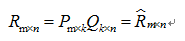其中，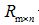代表原始的用户-商品矩阵，如下表3，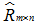代表对用户没有评价的商品进行推荐打分后的用户-商品矩阵，如下表4，k是可调参数。更新公式如下：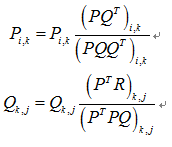损失函数：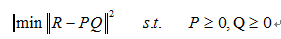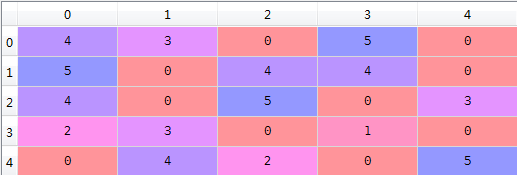表3 用户-商品矩阵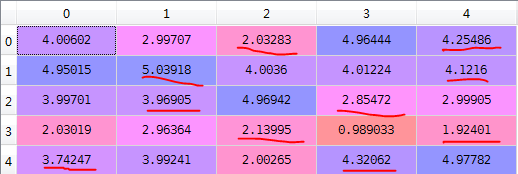表4 用户-商品矩阵（推荐表）# coding:UTF-8'''@author: zhaozhiyongDate:20160928'''import numpy as npdef load_data(path):    '''导入数据    output: data(mat):用户商品矩阵    input:  path(string):用户商品矩阵存储的位置    '''    f = open(path)        lines = line.strip().split("\t")    data = []    for line in f.readlines():        arr = []                arr.append(float(x))        for x in lines:            if x != "-":            else:    return np.mat(data)                arr.append(float(0))        data.append(arr)    input:  dataMat(mat):用户商品矩阵    f.close()
def gradAscent(dataMat, k, alpha, beta, maxCycles):    '''利用梯度下降法对矩阵进行分解            k(int):分解矩阵的参数    m, n = np.shape(dataMat)            alpha(float):学习率            beta(float):正则化参数            maxCycles(int):最大迭代次数    output: p,q(mat):分解后的矩阵    for step in range(maxCycles):    '''    # 1、初始化p和q    p = np.mat(np.random.random((m, k)))    q = np.mat(np.random.random((k, n)))
for r in range(k):    # 2、开始训练        for i in range(m):            for j in range(n):                if dataMat[i, j] > 0:                    error = dataMat[i, j]                        error = error - p[i, r] * q[r, j]                        q[r, j] = q[r, j] + alpha * (2 * error * p[i, r] - beta * q[r, j])                    for r in range(k):                        # 梯度上升                        p[i, r] = p[i, r] + alpha * (2 * error * q[r, j] - beta * p[i, r])        loss = 0.0        for i in range(m):                    # 3、计算损失函数            for j in range(n):                if dataMat[i, j] > 0:                    error = 0.0                    for r in range(k):                        error = error + p[i, r] * q[r, j]            break                    loss = (dataMat[i, j] - error) * (dataMat[i, j] - error)                    for r in range(k):                        loss = loss + beta * (p[i, r] * p[i, r] + q[r, j] * q[r, j]) / 2        if loss < 0.001:        if step % 1000 == 0:    for i in range(m):            print ("\titer: ", step, " loss: ", loss)    return p, q
def save_file(file_name, source):            source(mat):需要保存的文件    '''保存结果    input:  file_name(string):需要保存的文件名    '''
tmp = []    f = open(file_name, "w")    m, n = np.shape(source)
'''        for j in range(n):
tmp.append(str(source[i, j]))        f.write("\t".join(tmp) + "\n")def prediction(dataMatrix, p, q, user):    f.close()
'''为用户user未互动的项打分            p(mat):分解后的矩阵p    input:  dataMatrix(mat):原始用户商品矩阵            q(mat):分解后的矩阵q
k(int):推荐的商品个数            user(int):用户的id    output: predict(list):推荐列表    n = np.shape(dataMatrix)    predict = {}        if dataMatrix[user, j] == 0:    for j in range(n):            predict[j] = (p[user,] * q[:,j])[0,0]        # 按照打分从大到小排序def top_k(predict, k):    return sorted(predict.items(), key=lambda d:d, reverse=True)
'''为用户推荐前k个商品
print ("----------- 1、load data -----------")    input:  predict(list):排好序的商品列表    output: top_recom(list):top_k个商品    len_result = len(predict)    '''    top_recom = []        top_recom = predict    if k >= len_result:    else:
top_recom.append(predict[i])        for i in range(k):    return top_recom

dataMatrix = load_data("data.txt")
if __name__ == "__main__":
# 2、利用梯度下降法对矩阵进行分解    # 1、导入用户商品矩阵
print ("----------- 5、top_k recommendation ------------")    print ("----------- 2、training -----------")    p, q = gradAscent(dataMatrix, 10, 0.0002, 0.02, 10000)    # 3、保存分解后的结果    save_file("p", p)    print ("----------- 3、save decompose -----------")    print ("----------- 4、prediction -----------")    save_file("q", q)    # 4、预测
top_recom = top_k(predict, 2)    predict = prediction(dataMatrix, p, q, 0)    # 进行Top-K推荐
print (a)    print (top_recom)    a = p*q# coding:UTF-8
import numpy as npfrom mf import load_data, save_file, prediction, top_kdef train(V, r, maxCycles, e):    m, n = np.shape(V)    H = np.mat(np.random.random((r, n)))    # 1、初始化矩阵    W = np.mat(np.random.random((m, r)))            V_pre = W * H    # 2、非负矩阵分解    for step in range(maxCycles):        E = V - V_pre            for j in range(n):        err = 0.0        for i in range(m):                err += E[i, j] * E[i, j]            print ("\titer: ", step, " loss: " , err)        if err < e:            break        if step % 1000 == 0:        a = W.T * V                if b[i_1, j_1] != 0:        b = W.T * W * H        for i_1 in range(r):            for j_1 in range(n):        d = W * H * H.T                    H[i_1, j_1] = H[i_1, j_1] * a[i_1, j_1] / b[i_1, j_1]        c = V * H.T        for i_2 in range(m):    # 1、导入用户商品矩阵            for j_2 in range(r):                if d[i_2, j_2] != 0:                    W[i_2, j_2] = W[i_2, j_2] * c[i_2, j_2] / d[i_2, j_2]    return W, H

if __name__ == "__main__":    # 3、保存分解后的结果    print ("----------- 1、load data -----------")    V = load_data("data.txt")    # 2、非负矩阵分解
print ("----------- 2、training -----------")        W, H = train(V, 5, 10000, 1e-5)    # 进行Top-K推荐    print ("----------- 3、save decompose -----------")    save_file("W", W)    save_file("H", H)
predict = prediction(V, W, H, 0)    # 4、预测
print ("----------- 4、prediction -----------")
print (a)    print ("----------- 5、top_k recommendation ------------")    top_recom = top_k(predict, 2)    print (top_recom)
a = W * H

展开全文• 基于矩阵分解的CF算法 矩阵分解发展史 Traditional SVD: 通常SVD矩阵分解指的是SVD（奇异值）分解技术，在这我们姑且将其命名为Traditional SVD（传统并经典着）其公式如下： [外链图片转存失败,源站可能有防盗链...
基于矩阵分解的CF算法
矩阵分解发展史
Traditional SVD:
通常SVD矩阵分解指的是SVD（奇异值）分解技术，在这我们姑且将其命名为Traditional SVD（传统并经典着）其公式如下：
[外链图片转存失败,源站可能有防盗链机制,建议将图片保存下来直接上传(img-syiWJK8x-1596195407523)(img/矩阵分解1.jpg)]
Traditional SVD分解的形式为3个矩阵相乘，中间矩阵为奇异值矩阵。如果想运用SVD分解的话，有一个前提是要求矩阵是稠密的，即矩阵里的元素要非空，否则就不能运用SVD分解。
很显然我们的数据其实绝大多数情况下都是稀疏的，因此如果要使用Traditional SVD，一般的做法是先用均值或者其他统计学方法来填充矩阵，然后再运用Traditional SVD分解降维，但这样做明显对数据的原始性造成一定影响。
FunkSVD（LFM）
刚才提到的Traditional SVD首先需要填充矩阵，然后再进行分解降维，同时存在计算复杂度高的问题，因为要分解成3个矩阵，所以后来提出了Funk SVD的方法，它不在将矩阵分解为3个矩阵，而是分解为2个用户-隐含特征，项目-隐含特征的矩阵，Funk SVD也被称为最原始的LFM模型
[外链图片转存失败,源站可能有防盗链机制,建议将图片保存下来直接上传(img-syvFSBWa-1596195407528)(img/矩阵分解2.jpg)]
借鉴线性回归的思想，通过最小化观察数据的平方来寻求最优的用户和项目的隐含向量表示。同时为了避免过度拟合（Overfitting）观测数据，又提出了带有L2正则项的FunkSVD，上公式：
[外链图片转存失败,源站可能有防盗链机制,建议将图片保存下来直接上传(img-FR2zF1bD-1596195407536)(img/矩阵分解3.jpg)]
以上两种最优化函数都可以通过梯度下降或者随机梯度下降法来寻求最优解。
BiasSVD:
在FunkSVD提出来之后，出现了很多变形版本，其中一个相对成功的方法是BiasSVD，顾名思义，即带有偏置项的SVD分解：
[外链图片转存失败,源站可能有防盗链机制,建议将图片保存下来直接上传(img-CfTCF4Dc-1596195407539)(img/矩阵分解4.jpg)]
它基于的假设和Baseline基准预测是一样的，但这里将Baseline的偏置引入到了矩阵分解中
SVD++:
人们后来又提出了改进的BiasSVD，被称为SVD++，该算法是在BiasSVD的基础上添加了用户的隐式反馈信息：
[外链图片转存失败,源站可能有防盗链机制,建议将图片保存下来直接上传(img-8DPae9wN-1596195407543)(img/矩阵分解5.jpg)]
显示反馈指的用户的评分这样的行为，隐式反馈指用户的浏览记录、购买记录、收听记录等。
SVD++是基于这样的假设：在BiasSVD基础上，认为用户对于项目的历史浏览记录、购买记录、收听记录等可以从侧面反映用户的偏好。


展开全文• 我们知道有基于用户和基于物品的协同过滤算法，通过计算用户或物品的相似度来求得所缺失的打分。这里将潜在因子模型方法。 用户和物品分布在一个K维的特征空间，用户的未知评分可以简单的通过相应的用户和物品
• 　在讲SVD++之前，我还是想先回到基于物品相似的协同过滤算法。这个算法基本思想是找出一个用户有过正反馈的物品的相似的物品来给其作为推荐。其公式为： 　 　其中 rui 表示预测用户u对物品i的喜爱程度。wij 是...
• #!/usr/bin/env python # -*- coding: utf-8 -*- """ matrix_based CF """ import numpy as np ... def __init__(self, num_feature, num_recommendation): ...python svd
• Apr 08, 2014Categories intutorialtagged withMahouthadoop协同过滤Joe Jiang 前言：之前配置Mahout时测试过一个简单推荐例子，当时是在Eclipse上运行，由于集成插件缘故，所以一切进行都比较顺利，...
• 基于Laplace分布稀疏概率矩阵分解用于协同过滤研究论文
• 针对传统协同过滤算法普遍存在的稀疏性和冷启动问题，提出一种基于信任和矩阵分解的协同过滤推荐算法。提出一种基于用户评分值的隐式信任计算方法，该方法综合考虑用户的相似性和交互经验，运用信任传播方法使不存在...
• 基于矩阵分解的协同推荐采用一个线性模型表示用户对项目的评分。假定有若干个隐含分类特征，用户对某个项目的评分通过该项目归属于每个隐含分类特征的程度和用户对每个隐含分类特征的偏好程度的线性组合来体现。具体...
• 1.不同数据集大小对于矩阵分解算法有什么影响吗？ 或者下面这个表格空着一栏怎么填？ ![图片说明](https://img-ask.csdn.net/upload/202001/17/1579224905_741341.png) eg:UserCF描述我主要是针对...搜索引擎
• 在新手接触推荐系统这个领域时，遇到第一个理解起来比较困难就是协同过滤法。那么如果这时候百度话，得到最多是奇异值分解法，即（SVD）。SVD作用大致是将一个矩阵分解为三个矩阵相乘形式。如果运用在推荐...
• 本项目是一个电影推荐系统的项目，使用两种方式来实现： 基于CNN的推荐。 基于矩阵分解的协同过滤的推荐。代码相对于慕课网的代码有一些更新，大家以这里的代码为准。
• Transfer Learning for Collaborative Filtering via a Rating-Matrix Generative Model迁移学习
• 1. 引言 ...协同过滤推荐是其中运用最为成功技术之一。其中,基于用户最近邻法根据相似用户评分来预测当前用户评分。然而,在用户数量以及用户评分不足情况下,该方法存在冷启动和数据稀疏...spark 机器学习 推荐系统 最小二乘法
• 近年来,矩阵分解的协同过滤算法得到了煤炭销售系统的广泛应用。但是,随着互联网的快速发展,煤炭电子销售记录呈现指数级增长,传统的基于矩阵分解的协同聚类算法不能有效、快速地完成销售系统的推荐工作。文章针对大......